## Selina Concise Mathematics Class 10 ICSE Solutions Chapter 7 Ratio and Proportion (Including Properties and Uses) Ex 7D

These Solutions are part of Selina Concise Mathematics Class 10 ICSE Solutions. Here we have given Selina Concise Mathematics Class 10 ICSE Solutions Chapter 7 Ratio and Proportion Ex 7D.

Other Exercises

Question 1.
If a : b = 3 : 5, find : (10a + 3b) : (5a + 2b)
Solution:Question 2.
If 5x + 6y : 8x + 5y = 8 : 9, find x : y
Solution: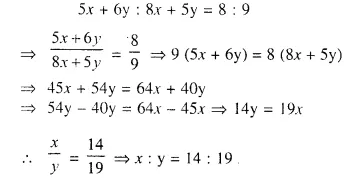Question 3.
If k (3x – 4y) : (2x – 3y) = (5x – 6y) : (4x – 5y), find x : y.
Solution: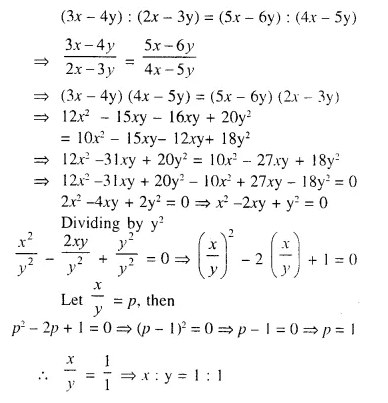Question 4.
Find the :
(i) duplicate ratio of 2√2 : 3√5
(ii) triplicate ratio of 2a : 3b,
(iii) sub-duplicate ratio of 9x2 a4 : 25y6 b2
(iv) sub-triplicate ratio of 216 : 343
(v) reciprocal ratio of 3 : 5
(vi) ratio compounded of the duplicate ratio of 5 : 6, the reciprocal ratio of 25 : 42 and the sub-duplicate ratio of 36 : 49.
Solution:Question 5.
Find the value of x, if :
(i) (2x + 3) : (5x – 38) is the duplicate ratio of √5 : √6
(ii) (2x + 1) : (3x + 13) is the sub-duplicate ratio of 9 : 25.
(iii) (3x -7): (4x + 3) is the sub-triplicate ratio of 8 : 27.
Solution: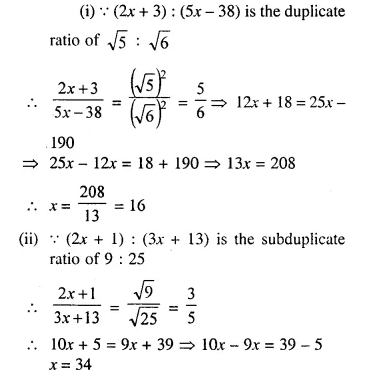Question 6.
What quantity must be added to each term of the ratio x : y so that it may become equal to c : d ?
Solution:Question 7.
A woman reduces her weight in the ratio 7 : 5. What does her weight become if originally it was 84 kg?
Solution:
Ratio in reduction of weight = 7 : 5
Originally weight of the woman = 84 kg
Reduced weight = $$\frac { 84 x 5 }{ 7 }$$ = 60 kg

Question 8.
If 15 (2x² – y²) = 7xy, find x : y, if x and y both are positive.
Solution: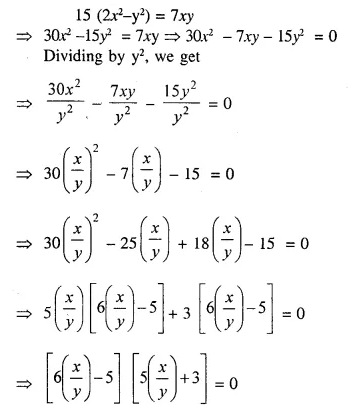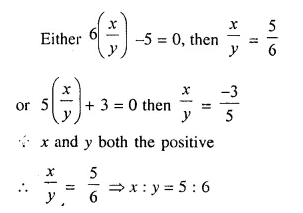Question 9.
Find the :
(i) fourth proportional to 2xy, x² and y².
(ii) third proportional to a² – b² and a + b.
(iii) mean proportion to (x – y) and (x3 – x²y)
Solution:
(i) Let a be the fourth proportional
Then 2xy : x² :: y² : a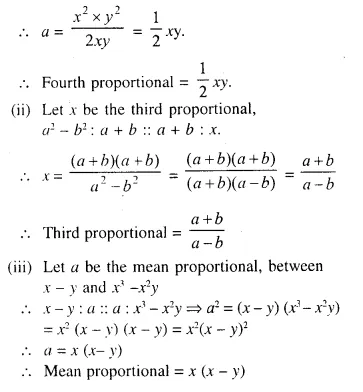Question 10.
Find two numbers such that the mean proportional between them is 14 and third proportional to them is 112.
Solution:
Let x and y be the two numbers.
Then 14 is the mean proportional between x and y
xy = 14² => xy = 196 ….(i)
and 112 is the third proportional to x, y
y² = 112 x ….(ii)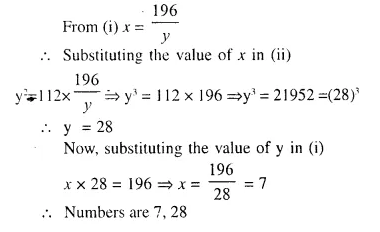Question 11.
If x and y be unequal and x : y is the duplicate ratio of x + z and y + z, prove that z is mean proportional between x and y.
Solution:Question 12.
If q is the mean proportional between p and r, prove that: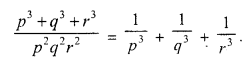Solution: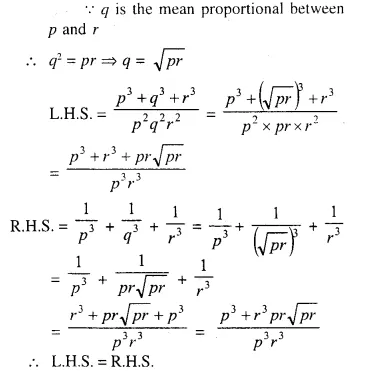Question 13.
If a, b and c are in continued proportion, prove that: a : c = (a² + b²) : (b² + c²).
Solution: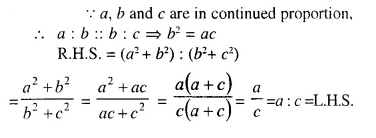Question 14.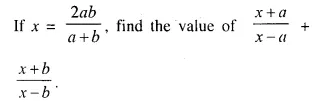Solution: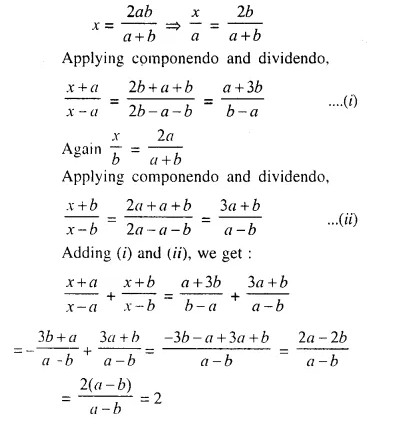Question 15.
If (4a + 9b) (4c – 9d) = (4a – 9b) (4c + 9d), prove that a : b = c : d.
Solution:Question 16.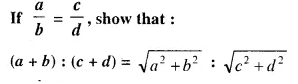Solution:Question 17.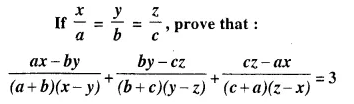Solution:Question 18.
There are 36 members in a student council in a school and the ratio of the number of boys to the number of girls is 3 : 1. How many more girls should be added to the council so that the ratio of number of boys to the number of girls may be 9 : 5 ?
Solution:
Total number of members = 36
Ratio in boys and girls = 3 : 1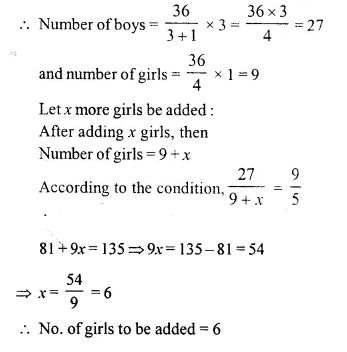Question 19.
If 7x – 15y = 4x + y, find the value of x : y. Hence, use componendo and dividendo to find the values of:Solution: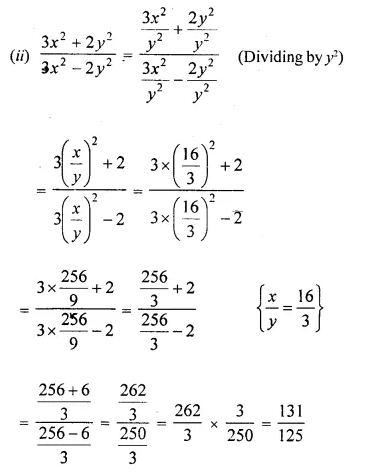Question 20.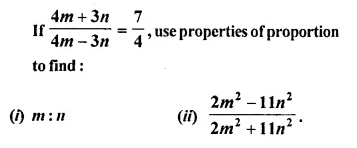Solution: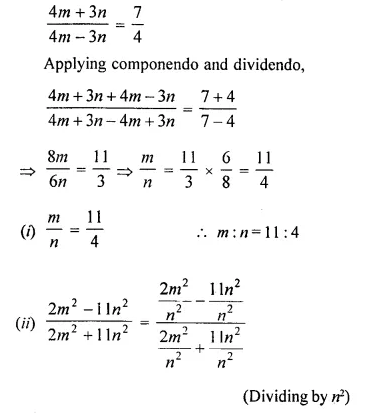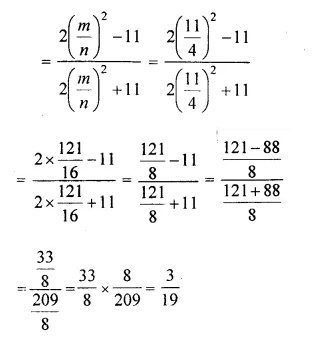Question 21.
If x, y, z arc in continued proportion, prove thatSolution: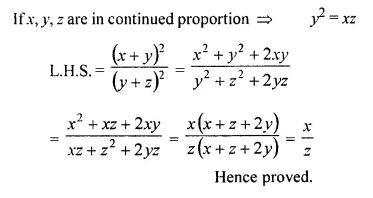Question 22.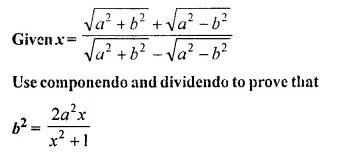Solution: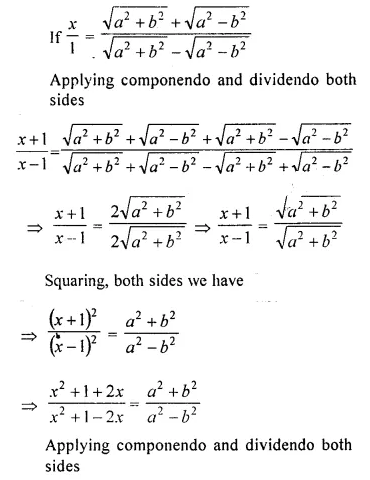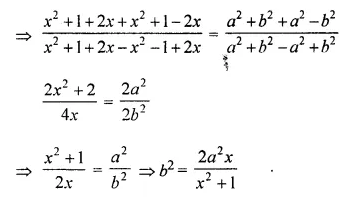Question 23.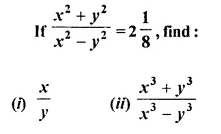Solution:Question 24.
Using componendo and dividendo, find the valueSolution: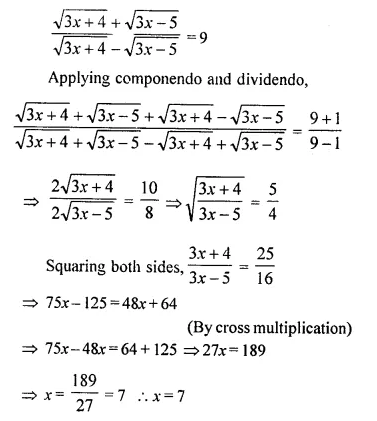Question 25.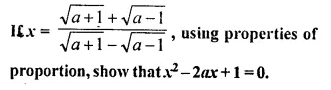Solution:Question 26.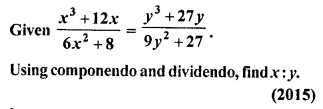Solution: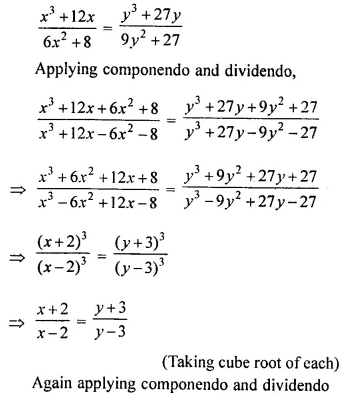Question 27.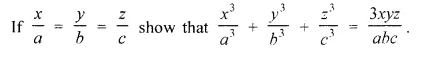Solution: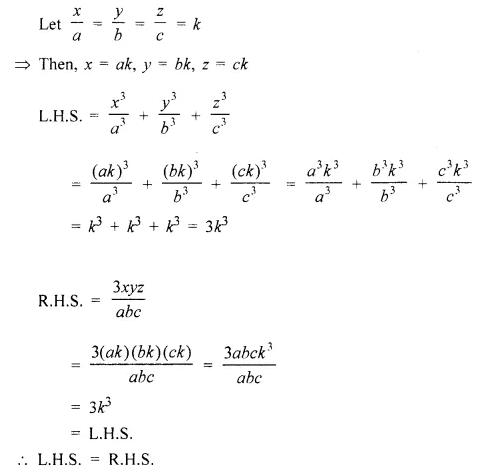Hope given Selina Concise Mathematics Class 10 ICSE Solutions Chapter 7 Ratio and Proportion Ex 7D are helpful to complete your math homework.

If you have any doubts, please comment below. Learn Insta try to provide online math tutoring for you.